Doppler effect

﻿
Doppler effectChange of wavelength caused by motion of the source.An animation illustrating how the Doppler effect causes a car engine or siren to sound higher in pitch when it is approaching than when it is receding. The pink circles are sound waves. When the car is moving to the left, each successive wave is emitted from a position further to the left than the previous wave. So for an observer in front (left) of the car, each wave takes slightly less time to reach him than the previous wave. The waves "bunch together", so the time between arrival of successive wavefronts is reduced, giving them a higher frequency. For an observer in back (right) of the car, each wave takes a slightly longer time to reach him than the previous wave. The waves "stretch apart", so the time between the arrival of successive wave-fronts is increased slightly, giving them a lower frequency.Doppler effect of water flow around a swan.

The Doppler effect (or Doppler shift), named after Austrian physicist Christian Doppler who proposed it in 1842 in Prague, is the change in frequency of a wave for an observer moving relative to the source of the wave. It is commonly heard when a vehicle sounding a siren or horn approaches, passes, and recedes from an observer. The received frequency is higher (compared to the emitted frequency) during the approach, it is identical at the instant of passing by, and it is lower during the recession.

The relative changes in frequency can be explained as follows. When the source of the waves is moving toward the observer, each successive wave crest is emitted from a position closer to the observer than the previous wave. Therefore each wave takes slightly less time to reach the observer than the previous wave. Therefore the time between the arrival of successive wave crests at the observer is reduced, causing an increase in the frequency. While they are travelling, the distance between successive wave fronts is reduced; so the waves "bunch together". Conversely, if the source of waves is moving away from the observer, each wave is emitted from a position farther from the observer than the previous wave, so the arrival time between successive waves is increased, reducing the frequency. The distance between successive wave fronts is increased, so the waves "spread out".

For waves that propagate in a medium, such as sound waves, the velocity of the observer and of the source are relative to the medium in which the waves are transmitted. The total Doppler effect may therefore result from motion of the source, motion of the observer, or motion of the medium. Each of these effects are analyzed separately. For waves which do not require a medium, such as light or gravity in general relativity, only the relative difference in velocity between the observer and the source needs to be considered.

Development

Doppler first proposed the effect in 1842 in his treatise "Über das farbige Licht der Doppelsterne und einiger anderer Gestirne des Himmels" (On the coloured light of the binary stars and some other stars of the heavens). The hypothesis was tested for sound waves by Buys Ballot in 1845. He confirmed that the sound's pitch was higher than the emitted frequency when the sound source approached him, and lower than the emitted frequency when the sound source receded from him. Hippolyte Fizeau discovered independently the same phenomenon on electromagnetic waves in 1848 (in France, the effect is sometimes called "l'effet Doppler-Fizeau" but that name was not adopted by the rest of the world as Fizeau's discovery was three years after Doppler's). In Britain, John Scott Russell made an experimental study of the Doppler effect (1848).

An English translation of Doppler's 1842 treatise can be found in the book 'The Search for Christian Doppler by Alec Eden.

GeneralStationary sound source produces sound waves at a constant frequency f, and the wave-fronts propagate symmetrically away from the source at a constant speed c (assuming speed of sound, c = 330 m/s), which is the speed of sound in the medium. The distance between wave-fronts is the wavelength. All observers will hear the same frequency, which will be equal to the actual frequency of the source where f = f0The same sound source is radiating sound waves at a constant frequency in the same medium. However, now the sound source is moving to the right with a speed υs = 0.7 c (Mach 0.7). The wave-fronts are produced with the same frequency as before. However, since the source is moving, the centre of each new wavefront is now slightly displaced to the right. As a result, the wave-fronts begin to bunch up on the right side (in front of) and spread further apart on the left side (behind) of the source. An observer in front of the source will hear a higher frequency$f = \left( \frac{c + v_r}{c - v_{s}} \right) f_0 = 3.33f_0\,$, and an observer behind the source will hear a lower frequency$f = \left( \frac{c - v_r}{c + v_{s}} \right) f_0 = 0.59f_0\,$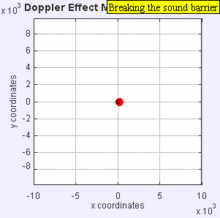Now the source is moving at the speed of sound in the medium (υs = c, or Mach 1). assuming the speed of sound in air at sea level is about 330 m/s . The wave fronts in front of the source are now all bunched up at the same point. As a result, an observer in front of the source will detect nothing until the source arrives where$f = \left( \frac{c + v_r}{c - v_{s}} \right) f_0 = \infty Hz\,$. An observer behind of the source$f = \left( \frac{c - v_r}{c + v_{s}} \right) f_0 = 0.5f_0\,$.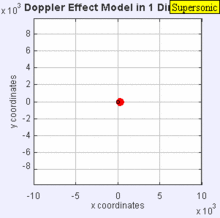The sound source has now broken through the sound speed barrier, and is traveling at 1.4 times the speed of sound, c (Mach 1.4). Since the source is moving faster than the sound waves it creates, it actually leads the advancing wavefront. The sound source will pass by a stationary observer before the observer actually hears the sound it creates. As a result, an observer in front of the source will detect$f = \left( \frac{c + v_r}{c - v_{s}} \right) f_0 = \infty Hz\,$ and an observer behind the source$f = \left( \frac{c - v_r}{c + v_{s}} \right) f_0 = 0.42f_0\,$.

In classical physics, where the speeds of source and the receiver relative to the medium are lower than the velocity of waves in the medium, the relationship between observed frequency f and emitted frequency f0 is given by:$f = \left( \frac{c \pm v_r}{c \mp v_{s}} \right) f_0 \,$
where$c \;$ is the velocity of waves in the medium$v_{r} \,$ is the velocity of the receiver relative to the medium; positive if the receiver is moving towards the source.$v_{s} \,$ is the velocity of the source relative to the medium; positive if the source is moving away from the receiver.

The frequency is decreased if either is moving away from the other.

The above formula works for sound waves if and only if the speeds of the source and receiver relative to the medium are slower than the speed of sound. See also Sonic boom.

The above formula assumes that the source is either directly approaching or receding from the observer. If the source approaches the observer at an angle (but still with a constant velocity), the observed frequency that is first heard is higher than the object's emitted frequency. Thereafter, there is a monotonic decrease in the observed frequency as it gets closer to the observer, through equality when it is closest to the observer, and a continued monotonic decrease as it recedes from the observer. When the observer is very close to the path of the object, the transition from high to low frequency is very abrupt. When the observer is far from the path of the object, the transition from high to low frequency is gradual.

In the limit where the speed of the wave is much greater than the relative speed of the source and observer (this is often the case with electromagnetic waves, e.g. light), the relationship between observed frequency f and emitted frequency f0 is given by:

Observed frequency Change in frequency$f=\left(1-\frac{v_{s,r}}{c}\right)f_0$$\Delta f=-\frac{v_{s,r}}{c}f_0=-\frac{v_{s,r}}{\lambda_{0}}$
where$v_{s,r} = v_s - v_r \,$ is the velocity of the source relative to the receiver: it is positive when the source and the receiver are moving further apart.$c \,$ is the speed of wave (e.g. 3×108 m/s for electromagnetic waves travelling in a vacuum)$\lambda_{0} \,$ is the wavelength of the transmitted wave in the reference frame of the source.

These two equations are only accurate to a first order approximation. However, they work reasonably well when the speed between the source and receiver is slow relative to the speed of the waves involved and the distance between the source and receiver is large relative to the wavelength of the waves. If either of these two approximations are violated, the formulae are no longer accurate.

Analysis

The frequency of the sounds that the source emits does not actually change. To understand what happens, consider the following analogy. Someone throws one ball every second in a man's direction. Assume that balls travel with constant velocity. If the thrower is stationary, the man will receive one ball every second. However, if the thrower is moving towards the man, he will receive balls more frequently because the balls will be less spaced out. The inverse is true if the thrower is moving away from the man. So it is actually the wavelength which is affected; as a consequence, the received frequency is also affected. It may also be said that the velocity of the wave remains constant whereas wavelength changes; hence frequency also changes.

If the source moving away from the observer is emitting waves through a medium with an actual frequency f0, then an observer stationary relative to the medium detects waves with a frequency f given by$f = \left ( \frac {c}{c + v_{s}} \right ) f_0$

where vs is positive if the source is moving away from the observer, and negative if the source is moving towards the observer.

A similar analysis for a moving observer and a stationary source yields the observed frequency (the receiver's velocity being represented as vr):$f = \left ( \frac {c + v_{r}}{c} \right ) f_0$

where the similar convention applies: vr is positive if the observer is moving towards the source, and negative if the observer is moving away from the source.

These can be generalized into a single equation with both the source and receiver moving.$f = \left ( \frac {c+v_{r}}{c + v_{s}} \right ) f_0$

With a relatively slow moving source, vs,r is small in comparison to v and the equation approximates to$f = \left (1 - \frac {v_{s,r}}{c} \right ) f_0$

where$v_{s,r}=v_s-v_r \,$.

However the limitations mentioned above still apply. When the more complicated exact equation is derived without using any approximations (just assuming that source, receiver, and wave or signal are moving linearly relatively to each other) several interesting and perhaps surprising results are found. For example, as Lord Rayleigh noted in his classic book on sound, by properly moving it would be possible to hear a symphony being played backwards. This is the so-called "time reversal effect" of the Doppler effect. Other interesting conclusions are that the Doppler effect is time-dependent in general (thus we need to know not only the source and receivers' velocities, but also their positions at a given time), and in some circumstances it is possible to receive two signals or waves from a source, or no signal at all. In addition there are more possibilities than just the receiver approaching the signal and the receiver receding from the signal.

All these additional complications are derived for the classical, i.e., non-relativistic, Doppler effect, but hold for the relativistic Doppler effect as well.[citation needed]

A common misconception

Craig Bohren pointed out in 1991 that some physics textbooks erroneously state that the observed frequency increases as the object approaches an observer and then decreases only as the object passes the observer. In most cases, the observed frequency of an approaching object declines monotonically from a value above the emitted frequency, through a value equal to the emitted frequency when the object is closest to the observer, and to values increasingly below the emitted frequency as the object recedes from the observer. Bohren proposed that this common misconception might occur because the intensity of the sound increases as an object approaches an observer and decreases once it passes and recedes from the observer and that this change in intensity is misperceived as a change in frequency. Higher sound pressure levels make for a small decrease in perceived pitch in low frequency sounds, and for a small increase in perceived pitch for high frequency sounds.

Application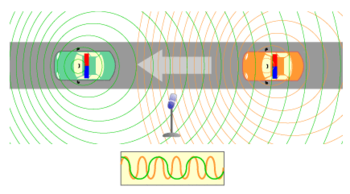A stationary microphone records moving police sirens at different pitches depending on their relative direction.

Sirens

The siren on a passing emergency vehicle will start out higher than its stationary pitch, slide down as it passes, and continue lower than its stationary pitch as it recedes from the observer. Astronomer John Dobson explained the effect thus:

"The reason the siren slides is because it doesn't hit you."

In other words, if the siren approached the observer directly, the pitch would remain constant (as vs, r is only the radial component) until the vehicle hit him, and then immediately jump to a new lower pitch. Because the vehicle passes by the observer, the radial velocity does not remain constant, but instead varies as a function of the angle between his line of sight and the siren's velocity:$v_{r}=v_s\cdot \cos{\theta}$

where vs is the velocity of the object (source of waves) with respect to the medium, and θ is the angle between the object's forward velocity and the line of sight from the object to the observer.

Astronomy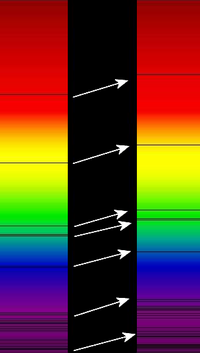Redshift of spectral lines in the optical spectrum of a supercluster of distant galaxies (right), as compared to that of the Sun (left).

The Doppler effect for electromagnetic waves such as light is of great use in astronomy and results in either a so-called red shift or blue shift. It has been used to measure the speed at which stars and galaxies are approaching or receding from us, that is, the radial velocity. This is used to detect if an apparently single star is, in reality, a close binary and even to measure the rotational speed of stars and galaxies.

The use of the Doppler effect for light in astronomy depends on our knowledge that the spectra of stars are not continuous. They exhibit absorption lines at well defined frequencies that are correlated with the energies required to excite electrons in various elements from one level to another. The Doppler effect is recognizable in the fact that the absorption lines are not always at the frequencies that are obtained from the spectrum of a stationary light source. Since blue light has a higher frequency than red light, the spectral lines of an approaching astronomical light source exhibit a blue shift and those of a receding astronomical light source exhibit a redshift.

Among the nearby stars, the largest radial velocities with respect to the Sun are +308 km/s (BD-15°4041, also known as LHS 52, 81.7 light-years away) and -260 km/s (Woolley 9722, also known as Wolf 1106 and LHS 64, 78.2 light-years away). Positive radial velocity means the star is receding from the Sun, negative that it is approaching.

Temperature measurement

Another use of the Doppler effect, which is found mostly in plasma physics and astronomy, is the estimation of the temperature of a gas (or ion temperature in a plasma) which is emitting a spectral line. Due to the thermal motion of the emitters, the light emitted by each particle can be slightly red- or blue-shifted, and the net effect is a broadening of the line. This line shape is called a Doppler profile and the width of the line is proportional to the square root of the temperature of the emitting species, allowing a spectral line (with the width dominated by the Doppler broadening) to be used to infer the temperature.

The Doppler effect is used in some types of radar, to measure the velocity of detected objects. A radar beam is fired at a moving target — e.g. a motor car, as police use radar to detect speeding motorists — as it approaches or recedes from the radar source. Each successive radar wave has to travel farther to reach the car, before being reflected and re-detected near the source. As each wave has to move farther, the gap between each wave increases, increasing the wavelength. In some situations, the radar beam is fired at the moving car as it approaches, in which case each successive wave travels a lesser distance, decreasing the wavelength. In either situation, calculations from the Doppler effect accurately determine the car's velocity. Moreover, the proximity fuze, developed during World War II, relies upon Doppler radar to explode at the correct time, height, distance, etc.[citation needed]

Medical imaging and blood flow measurement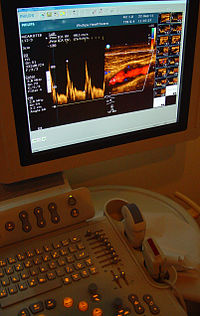colour flow ultrasonography (Doppler) of a carotid artery - scanner and screen

An echocardiogram can, within certain limits, produce accurate assessment of the direction of blood flow and the velocity of blood and cardiac tissue at any arbitrary point using the Doppler effect. One of the limitations is that the ultrasound beam should be as parallel to the blood flow as possible. Velocity measurements allow assessment of cardiac valve areas and function, any abnormal communications between the left and right side of the heart, any leaking of blood through the valves (valvular regurgitation), and calculation of the cardiac output. Contrast-enhanced ultrasound using gas-filled microbubble contrast media can be used to improve velocity or other flow-related medical measurements.

Although "Doppler" has become synonymous with "velocity measurement" in medical imaging, in many cases it is not the frequency shift (Doppler shift) of the received signal that is measured, but the phase shift (when the received signal arrives).

Velocity measurements of blood flow are also used in other fields of medical ultrasonography, such as obstetric ultrasonography and neurology. Velocity measurement of blood flow in arteries and veins based on Doppler effect is an effective tool for diagnosis of vascular problems like stenosis.

Flow measurement

Instruments such as the laser Doppler velocimeter (LDV), and acoustic Doppler velocimeter (ADV) have been developed to measure velocities in a fluid flows. The LDV emits a light beam and the ADV emits an ultrasonic acoustic burst, and measure the Doppler shift in wavelengths of reflections from particles moving with the flow. The actual flow is computed as a function of the water velocity and phase. This technique allows non-intrusive flow measurements, at high precision and high frequency.

Velocity profile measurement

Developed originally for velocity measurements in medical applications (blood flow), Ultrasonic Doppler Velocimetry (UDV) can measure in real time complete velocity profile in almost any liquids containing particles in suspension such as dust, gas bubbles, emulsions. Flows can be pulsating, oscillating, laminar or turbulent, stationary or transient. This technique is fully non-invasive.

Underwater acoustics

In military applications the Doppler shift of a target is used to ascertain the speed of a submarine using both passive and active sonar systems. As a submarine passes by a passive sonobuoy, the stable frequencies undergo a Doppler shift, and the speed and range from the sonobuoy can be calculated. If the sonar system is mounted on a moving ship or another submarine, then the relative velocity can be calculated.

Audio

The Leslie speaker, associated with and predominantly used with the Hammond B-3 organ, takes advantage of the Doppler Effect by using an electric motor to rotate an acoustic horn around a loudspeaker, sending its sound in a circle. This results at the listener's ear in rapidly fluctuating frequencies of a keyboard note.

Vibration measurement

A laser Doppler vibrometer (LDV) is a non-contact method for measuring vibration. The laser beam from the LDV is directed at the surface of interest, and the vibration amplitude and frequency are extracted from the Doppler shift of the laser beam frequency due to the motion of the surface.

Wikimedia Foundation. 2010.

Look at other dictionaries:

• Doppler effect — Doppler io efektas statusas T sritis automatika atitikmenys: angl. Doppler effect vok. Doppler Effekt, m rus. доплеровский эффект, m; эффект Доплера, m pranc. effet Doppler, m ryšiai: sinonimas – Doplerio efektas …   Automatikos terminų žodynas

• Doppler effect — Doppler io reiškinys statusas T sritis automatika atitikmenys: angl. Doppler effect vok. Doppler Effekt, m rus. доплеровский эффект, m pranc. effet Doppler, m ryšiai: sinonimas – Doplerio reiškinys …   Automatikos terminų žodynas

• Doppler effect — (also Doppler shift) ► NOUN Physics ▪ an increase (or decrease) in the apparent frequency of sound, light, or other waves as the source and the observer move towards (or away from) each other. ORIGIN named after the Austrian physicist Johann… …   English terms dictionary

• Doppler effect — [däp′lər] n. [after C. Doppler (1803 53), Austrian mathematician and physicist] the apparent change of frequency of sound waves or light waves, varying with the relative velocity of the source and the observer: if the source and observer are… …   English World dictionary

• Doppler effect — n a change in the frequency with which waves (as sound, light, or radio waves) from a given source reach an observer when the source and the observer are in motion with respect to each other so that the frequency increases or decreases according… …   Medical dictionary

• Doppler effect — /dop leuhr/, Physics. (often l.c.) the shift in frequency (Doppler shift) of acoustic or electromagnetic radiation emitted by a source moving relative to an observer as perceived by the observer: the shift is to higher frequencies when the source …   Universalium

• Doppler effect —    Also known as Doppler shift. Both eponyms refer to the Austrian mathematician and physicist Christian Andreas Doppler (1803 1853), who first described the effect in or shortly before 1842. In perceptual neuroscience, they are used to denote an …   Dictionary of Hallucinations

• Doppler effect — Doplerio efektas statusas T sritis Standartizacija ir metrologija apibrėžtis Matuojamosios spinduliuotės dažnio pokytis, atsirandantis dėl reliatyviojo judesio tarp pirminio ar antrinio šaltinio ir stebėtojo. atitikmenys: angl. Doppler effect vok …   Penkiakalbis aiškinamasis metrologijos terminų žodynas

• Doppler effect — Doplerio efektas statusas T sritis fizika atitikmenys: angl. Doppler effect vok. Doppler Effekt, m rus. эффект Доплера, m pranc. effet Doppler, m …   Fizikos terminų žodynas

• Doppler effect — Doplerio reiškinys statusas T sritis fizika atitikmenys: angl. Doppler effect vok. Doppler Effekt, m rus. эффект Доплера, m; явление Доплера, n pranc. effet Doppler, m …   Fizikos terminų žodynas